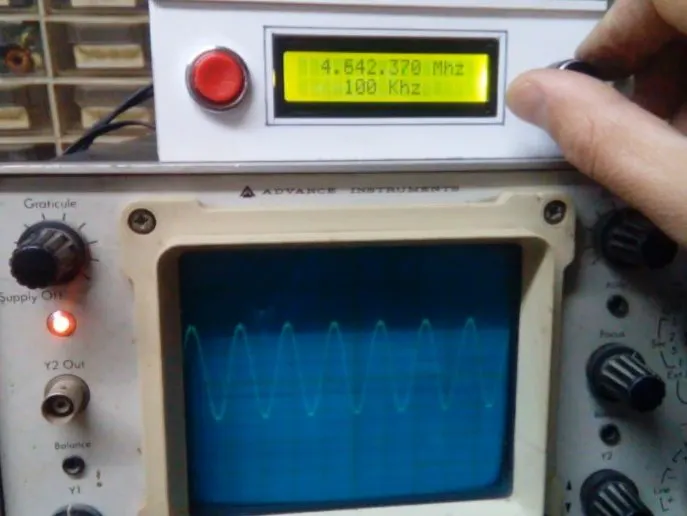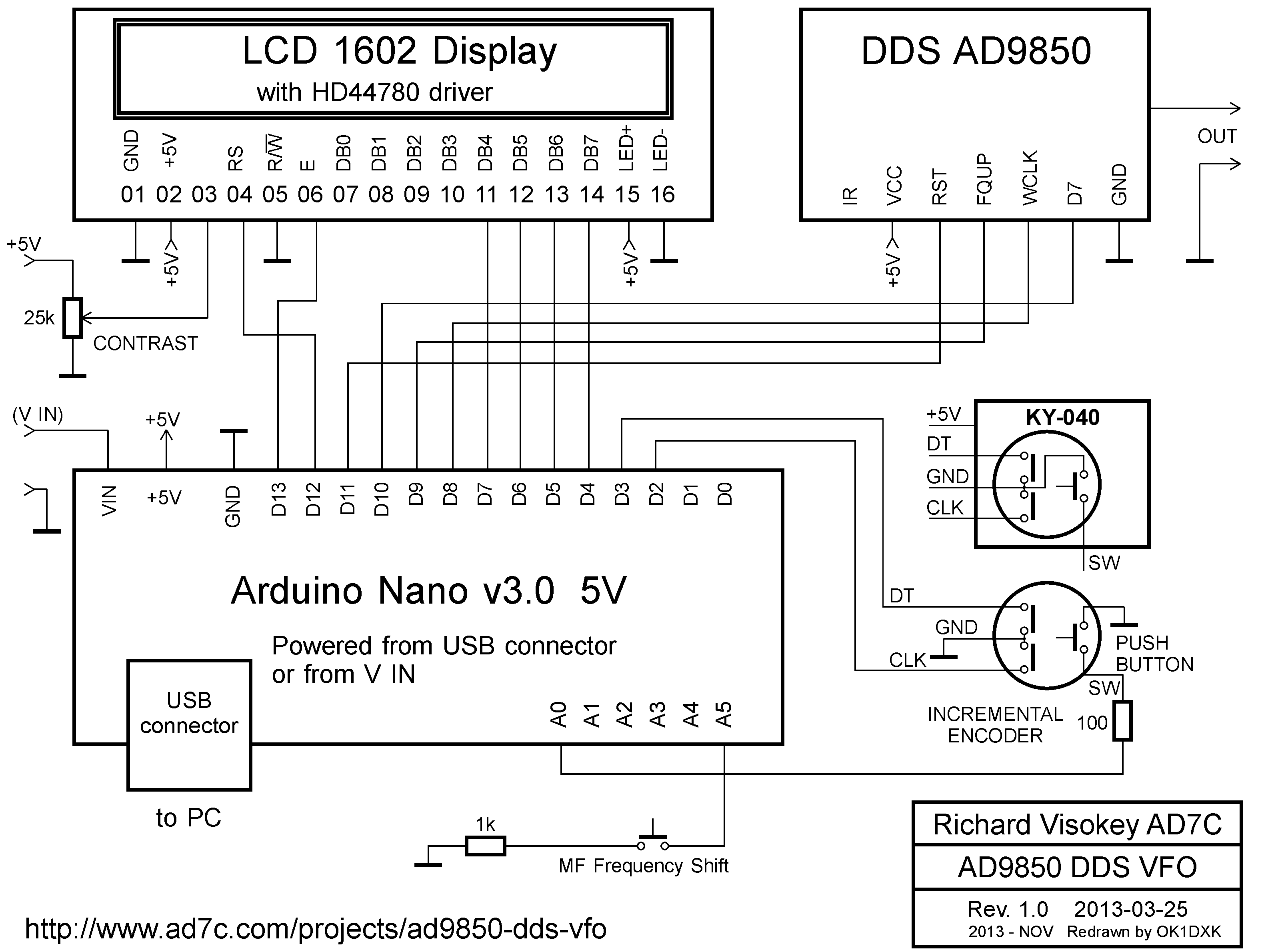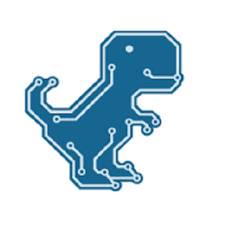Project tutorial# Arduino DDS VFO with AD9850 Module © GPL3+

Inexpensive to build but a very useful tool for every electronics laboratory.

• 32,359 views
• 22 respects

## Necessary tools and machinesSoldering iron (generic)

## Code

##### codeArduino
```/*
Main code by Richard Visokey AD7C - www.ad7c.com
Revision 2.0 - November 6th, 2013
*/

// Include the library code
#include <LiquidCrystal.h>
#include <rotary.h>
#include <EEPROM.h>

//Setup some items
#define W_CLK 8   // Pin 8 - connect to AD9850 module word load clock pin (CLK)
#define FQ_UD 9   // Pin 9 - connect to freq update pin (FQ)
#define DATA 10   // Pin 10 - connect to serial data load pin (DATA)
#define RESET 11  // Pin 11 - connect to reset pin (RST)
#define pulseHigh(pin) {digitalWrite(pin, HIGH); digitalWrite(pin, LOW); }
Rotary r = Rotary(2,3); // sets the pins the rotary encoder uses.  Must be interrupt pins.
LiquidCrystal lcd(12, 13, 7, 6, 5, 4); // I used an odd pin combination because I need pin 2 and 3 for the interrupts.
int_fast32_t rx=7150000; // Base (starting) frequency of VFO.  This only loads once.  To force load again see ForceFreq variable below.
int_fast32_t rx2=1; // variable to hold the updated frequency
int_fast32_t increment = 10; // starting VFO update increment in HZ.
int_fast32_t iffreq = 4192000; // Intermedite Frequency - Amount to subtract (-) from base frequency. ********************************************
int buttonstate = 0;
int buttonstate2 = 0;
int GoIF = 1;
String hertz = "10 Hz";
int  hertzPosition = 5;
byte ones,tens,hundreds,thousands,tenthousands,hundredthousands,millions ;  //Placeholders
String freq; // string to hold the frequency
int_fast32_t timepassed = millis(); // int to hold the arduino miilis since startup
int memstatus = 1;  // value to notify if memory is current or old. 0=old, 1=current.

int ForceFreq = 1;  // Change this to 0 after you upload and run a working sketch to activate the EEPROM memory.  YOU MUST PUT THIS BACK TO 0 AND UPLOAD THE SKETCH AGAIN AFTER STARTING FREQUENCY IS SET!

void setup() {
pinMode(A0,INPUT); // Connect to a button that goes to GND on push
pinMode(A5,INPUT); // IF sense **********************************************
digitalWrite(A0,HIGH);
digitalWrite(A5,HIGH);
lcd.begin(16, 2);
PCICR |= (1 << PCIE2);
PCMSK2 |= (1 << PCINT18) | (1 << PCINT19);
sei();
pinMode(FQ_UD, OUTPUT);
pinMode(W_CLK, OUTPUT);
pinMode(DATA, OUTPUT);
pinMode(RESET, OUTPUT);
pulseHigh(RESET);
pulseHigh(W_CLK);
pulseHigh(FQ_UD);  // this pulse enables serial mode on the AD9850 - Datasheet page 12.
lcd.setCursor(hertzPosition,1);
lcd.print(hertz);

// Load the stored frequency
if (ForceFreq == 0) {
rx = freq.toInt();
}
}

void loop() {
// Update the display and frequency if the new Freq NEQ the old Freq
if (rx != rx2){
showFreq();
sendFrequency(rx);
rx2 = rx;
}

// Rotate through the rate of tuning as you hold down the button
if(buttonstate == LOW) {
setincrement();
};

// Check for PIN low to drive IF offset Freq
if (buttonstate != buttonstate2){
if(buttonstate == LOW) {
lcd.setCursor(15,1);
lcd.print(".");
GoIF = 0;
buttonstate2 = buttonstate;
sendFrequency(rx);
}
else{
lcd.setCursor(15,1);
lcd.print(" ");
GoIF = 1;
buttonstate2 = buttonstate;
sendFrequency(rx);
};
};

// Write the frequency to memory if not stored and 2 seconds have passed since the last frequency change.
if(memstatus == 0){
if(timepassed+2000 < millis()){
storeMEM();
}
}

}

// Interrupt routine to catch the rotary encoder
ISR(PCINT2_vect) {
unsigned char result = r.process();
if (result) {
if (result == DIR_CW){rx=rx+increment;}
else {rx=rx-increment;};
if (rx >=30000000){rx=rx2;}; // UPPER VFO LIMIT
if (rx <=1000000){rx=rx2;}; // LOWER VFO LIMIT
}
}

// frequency calc from datasheet page 8 = <sys clock> * <frequency tuning word>/2^32
void sendFrequency(double frequency) {
if (GoIF == 1){frequency=frequency-iffreq;}; //If pin = low, subtract the IF frequency.
int32_t freq = frequency * 4294967295/125000000;  // note 125 MHz clock on 9850.  You can make 'slight' tuning variations here by adjusting the clock frequency.
for (int b=0; b<4; b++, freq>>=8) {
tfr_byte(freq & 0xFF);
}
tfr_byte(0x000);   // Final control byte, all 0 for 9850 chip
pulseHigh(FQ_UD);  // Done!  Should see output
}
// transfers a byte, a bit at a time, LSB first to the 9850 via serial DATA line
void tfr_byte(byte data)
{
for (int i=0; i<8; i++, data>>=1) {
digitalWrite(DATA, data & 0x01);
pulseHigh(W_CLK);   //after each bit sent, CLK is pulsed high
}
}

void setincrement(){
if(increment == 10){increment = 50; hertz = "50 Hz"; hertzPosition=5;}
else if (increment == 50){increment = 100;  hertz = "100 Hz"; hertzPosition=4;}
else if (increment == 100){increment = 500; hertz="500 Hz"; hertzPosition=4;}
else if (increment == 500){increment = 1000; hertz="1 Khz"; hertzPosition=6;}
else if (increment == 1000){increment = 2500; hertz="2.5 Khz"; hertzPosition=4;}
else if (increment == 2500){increment = 5000; hertz="5 Khz"; hertzPosition=6;}
else if (increment == 5000){increment = 10000; hertz="10 Khz"; hertzPosition=5;}
else if (increment == 10000){increment = 100000; hertz="100 Khz"; hertzPosition=4;}
else if (increment == 100000){increment = 1000000; hertz="1 Mhz"; hertzPosition=6;}
else{increment = 10; hertz = "10 Hz"; hertzPosition=5;};
lcd.setCursor(0,1);
lcd.print("                ");
lcd.setCursor(hertzPosition,1);
lcd.print(hertz);
delay(250); // Adjust this delay to speed up/slow down the button menu scroll speed.
};

void showFreq(){
millions = int(rx/1000000);
hundredthousands = ((rx/100000)%10);
tenthousands = ((rx/10000)%10);
thousands = ((rx/1000)%10);
hundreds = ((rx/100)%10);
tens = ((rx/10)%10);
ones = ((rx/1)%10);
lcd.setCursor(0,0);
lcd.print("                ");
if (millions > 9){lcd.setCursor(1,0);}
else{lcd.setCursor(2,0);}
lcd.print(millions);
lcd.print(".");
lcd.print(hundredthousands);
lcd.print(tenthousands);
lcd.print(thousands);
lcd.print(".");
lcd.print(hundreds);
lcd.print(tens);
lcd.print(ones);
lcd.print(" Mhz  ");
timepassed = millis();
memstatus = 0; // Trigger memory write
};

void storeMEM(){
//Write each frequency section to a EPROM slot.  Yes, it's cheating but it works!
EEPROM.write(0,millions);
EEPROM.write(1,hundredthousands);
EEPROM.write(2,tenthousands);
EEPROM.write(3,thousands);
EEPROM.write(4,hundreds);
EEPROM.write(5,tens);
EEPROM.write(6,ones);
memstatus = 1;  // Let program know memory has been written
};
```

## Schematics#### Author##### Mirko Pavleski
• 108 projects
• 641 followers

January 1, 2019

#### Members who respect this project

See similar projects
you might like

#### DIY Sensitive Software Defined Radio with AD9850 VFO

Project tutorial by Mirko Pavleski

• 14,125 views
• 22 respects

#### DIY Si4730 All Band Radio (LW, MW, SW, FM)

Project tutorial by Mirko Pavleski

• 51,123 views
• 80 respects

#### Soldering Iron Controller for Hakko 907

Project tutorial by Alexander

• 42,376 views
• 83 respects

#### DIY Retro Look FM Radio with TEA5767 Module

Project tutorial by Mirko Pavleski

• 31,317 views
• 72 respects

• 1,461 views